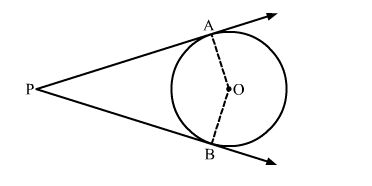# In the given figure, O is the centre of the circle. PA and PB are tangents.`
Question:

In the given figure, O is the centre of the circle. PA and PB are tangents. Show that AOBP is a cyclic quadrilateralSolution:

We know that the radius and tangent are perpendicular at their point of contact
∵∠OBP = ∠OAP = 90